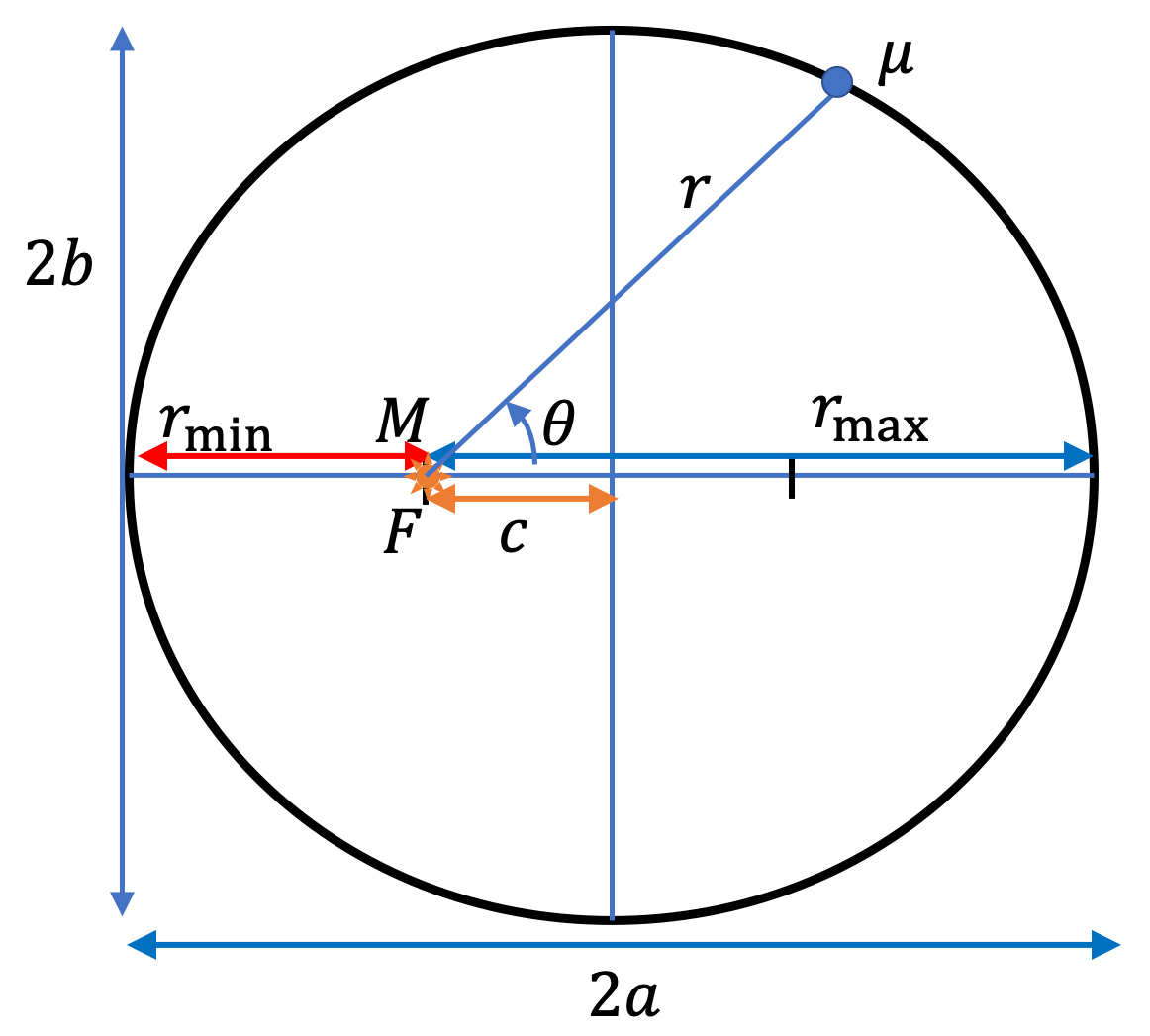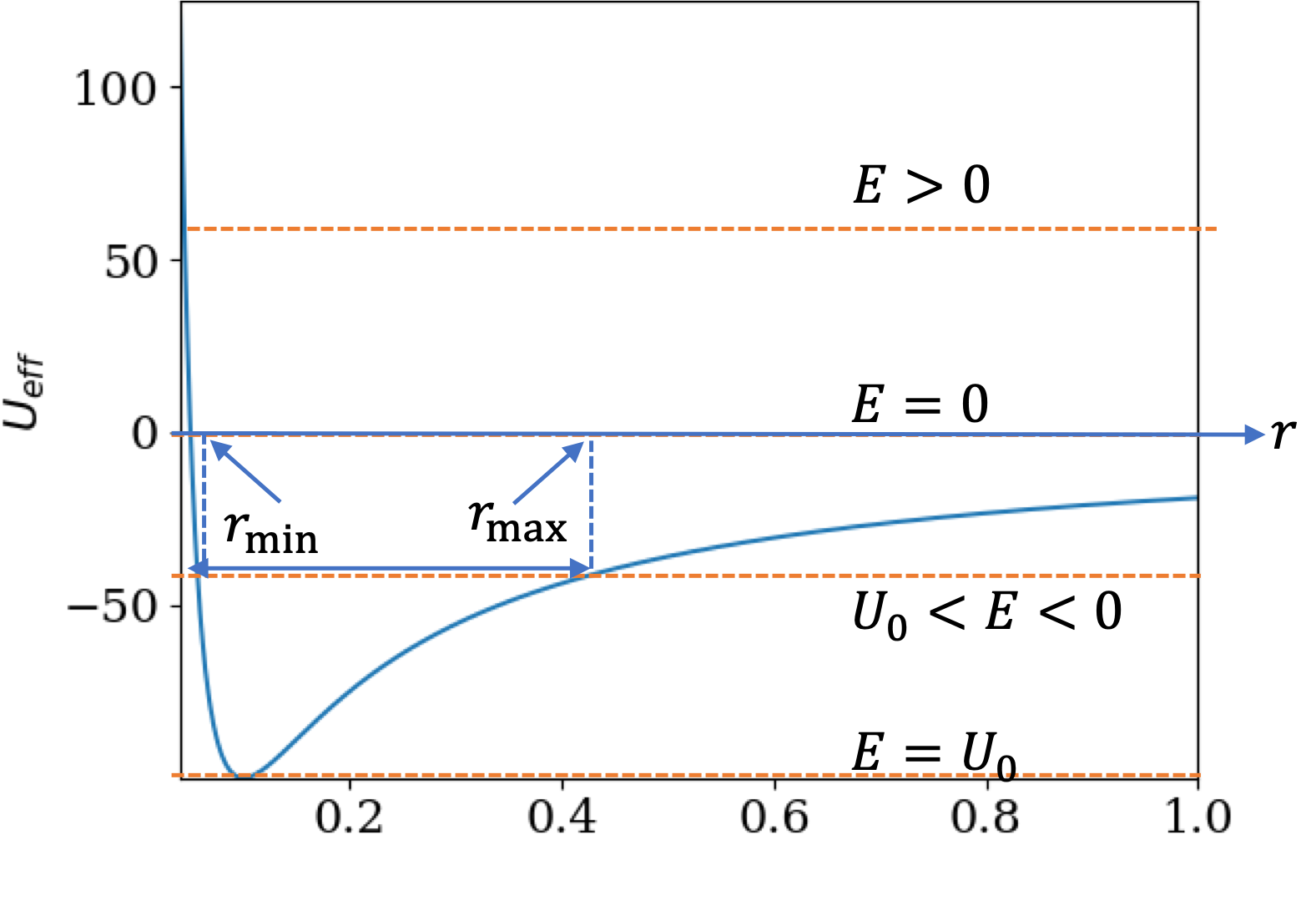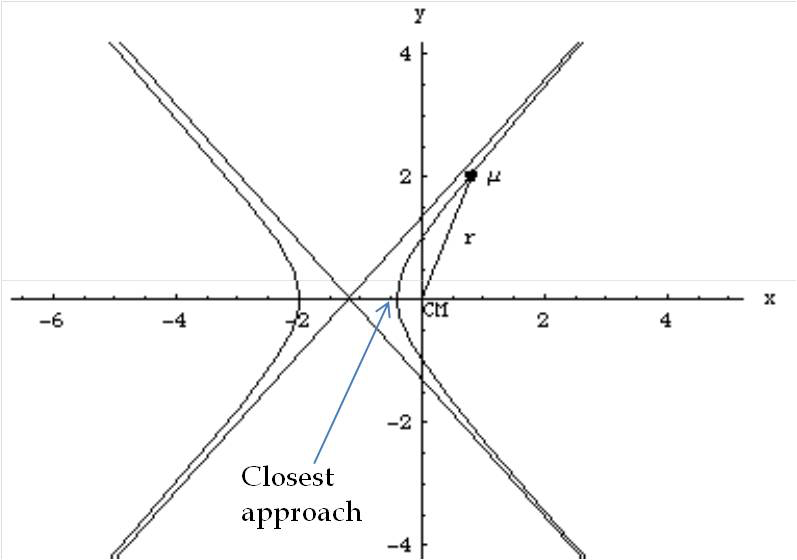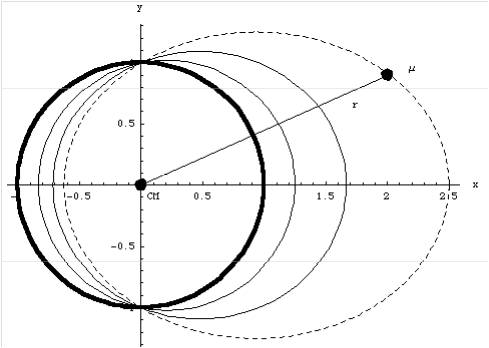## Section12.7The Orbit Equation

Orbit equation gives the analytic expression of the orbit of a planet in a planet-Sun two-body system. We usullay study these oribits in the CM frame, where the orbit equation refers to the orbit of the reduced mass $\mu$ about the origin, where we place total mass $M$ at rest. In this approach, we obtain evolution of relative motion of the planet with respect to Sun. The equation of motion will be derived in the Calculus section below.

I will state the final answer here so that we can discuss the physical consequences of the result, which are important for understanding the motion of planets. You may not be surprised that the solution of the equation of motion, Eq. (12.7.9) given below, is an ellipse, which in polar coordinates with origin at one of the foci of the ellipse is

\begin{equation} r = \frac{r_0}{1-e\cos\,\theta}.\label{eq-orbit-equation-solution}\tag{12.7.1} \end{equation}In terms of the masses, energy, and angular momentum, the parameters, $r_0$ and eccentricity $e\text{,}$ of the ellipse are

\begin{equation} r_0 = \dfrac{l^2}{G_N m_1m_2\mu}, \ \ \ e = \sqrt{1 + \dfrac{E}{|U_0|} },\label{eq-orbit-equation-parameters}\tag{12.7.2} \end{equation}

where, as before,

\begin{equation*} M = m_1 + m_2,\ \ \mu = \dfrac{m_1m_2}{M},\ \ \ |U_0| = \dfrac{(G_N m_1 m_2)^2 \mu}{2l^2}. \end{equation*}

The semi-major $a\text{,}$ semi-minor $b\text{,}$ and focal distance $c$ of the ellipse are

\begin{equation} a = \dfrac{r_0}{1-e^2},\ \ b = \dfrac{r_0}{\sqrt{1-e^2}},\ \ c = \dfrac{e\,r_0}{1-e^2}.\label{eq-orbit-eqn-ellipse-parameters}\tag{12.7.3} \end{equation}

### Subsection12.7.1Four Types of Orbits

Depending upon energy, we have seen four types of possible orbits. From Eq. (12.7.2) eccenetricity of orbit depends on energy. The part of energy that is other than the kinetic energy of radial motion, i.e., the term containing $(dr/dt)^2\text{,}$ is called effective potential energy, $U_\text{eff}\text{.}$ You can see that $U_\text{eff}$ is sum of regular potential energy and the angular motion part.

\begin{equation} U_\text{eff} = \frac{1}{2}\frac{l^2}{\mu r^2} - \frac{G_N m_1 m_2}{r}.\tag{12.7.4} \end{equation}

When you plot this with respect to $r\text{,}$ as shown in Figure 12.7.1, you find that, depending on energy $E\text{,}$ there are cases of one turning point and two turning points in the motion. In the case of two turning points, they corresponding to the minimum $r$ and maximum $r\text{.}$ These are perihelion and aphelion in the case of Sun-Planet system.Figure 12.7.1. The effective potential energy diagram shows four different types of motion depending upon the energy $E$ of the system. (1) Circular motion when $E = U_0\text{,}$ the minimum of $U_\text{eff}\text{,}$ (2) Elliptical motion when $U_0 \lt E \lt 0 \text{,}$ (3) Parabolic motion when $E=0\text{,}$ and (4) Elliptical motion when $E \gt 0\text{.}$

To discuss the type of orbits that result for various values of energy as compared to the minimum effective potential energy, it is best to rewrite the parameters $r_0$ and $e$ in terms of the minimum value of the effective potential energy $U^{\text{min}}_{\text{eff}}\text{,}$ which we will denote by symbol $U_0\text{.}$ By an elementary calculation, you can show that the minimum value of the effective potential energy is at

\begin{equation} U_0 = -\dfrac{(G_N m_1 m_2)^2 \mu}{2l^2}\tag{12.7.5} \end{equation}

Therefore, the parameters $r_0$ and $e$ in the orbit equation are

\begin{equation} r_0 = \frac{l}{\sqrt{-2\mu U_0}},\ \ \ \ e = \sqrt{1- \frac{E}{U_0}}.\tag{12.7.6} \end{equation}

Four Cases:

1. $E = 0.$

If $E = 0\text{,}$ then $e=1\text{.}$ The trajectory is not an ellipse, but a parabola.

2. $E \gt 0.$

If $E \gt 0\text{,}$ then $e \gt 1$ since $U_0 \lt 0\text{.}$ This corresponds to a hyperbolic trajectory. This type of object will get closest to the Sun only once. See Figure 12.7.2 for an example.

3. $U_0 \lt E \lt 0.$

This case corresponds to $0 \lt e \lt 1 \text{.}$ Hence, the orbit is an ellipse. This is the case for the bound planetary orbits about the Sun. See Figure 12.7.3 for an example.

4. $E=U_0.$

This makes $e = 0\text{.}$ With $e = 0\text{,}$ $r = r_0\text{.}$ Therefore, the trajectory is a circle of radius $r_0\text{.}$ The orbit of Earth is very close to this case.Figure 12.7.2. Hyperbolic orbit for $r_0 = 1$ unit and $e = 1.5$ for an attractive potential. The other branch is for a repulsive force since acceleration will be pointed away from the CM.Figure 12.7.3. Elliptical orbits for three eccentricities $0.2, 0.4, 0.6\text{.}$ The thick solid line is a circular orbit which corresponds to $e = 0\text{.}$ The higher the eccentricity more elongated the ellipse. The value of $r_0$ was set to 1 for the plots.

Both cases 3 and 4 correspond to bound orbits. Hence planets (as well as comets) have either elliptical or circular orbits around the Sun confirming Kepler's first law. The orbits of different planets differ in their eccentricities. The orbit of Venus is almost a circle while the orbit of Pluto is quite elongated. Comets have much higher eccentricities. Checkout eccentricities and other parameters of planets at the NASA website.

### Subsection12.7.2(Calculus) The Equation of Motion for Orbit Equation

To obtain the orbit of fictitious particle of reduced mass $\mu$ in a two-body system, it is sufficient to examine the conservation of angular momentum and the conservation of energy in the system, which we write in polar coordinates. Denote conserved angular momentum by $l$ and energy by $E\text{.}$

\begin{align} \amp \mu r^2 \dfrac{d\theta}{dt} = l,\label{cons-ang-mom-mu-1}\tag{12.7.7}\\ \amp \dfrac{1}{2}\mu\left( \dfrac{dr}{dt} \right)^2 + \frac{1}{2}\frac{l^2}{\mu r^2} - \frac{G_N m_1 m_2}{r} = E.\label{cons-enr-mu-1}\tag{12.7.8} \end{align}

Isolate the first term with $(dr/dt)^2$ in the second equation and divide this by the square of the first equation gives

\begin{equation} \dfrac{dr}{d\theta} = \pm \sqrt{ \alpha\, r^4 + \dfrac{2}{r_0}\, r^3 - 2 r^2 },\label{eq-orbit-equation-dr-dtheta}\tag{12.7.9} \end{equation}

where

\begin{equation*} \alpha = \dfrac{2 \mu\,E}{l^2},\ \ \dfrac{1}{r_0} = \dfrac{G_N M}{l^2}\ \ \text{with}\ \ M = m_1 + m_2. \end{equation*}

Integrating this equation will give the equation of the trajectory as the function $r(\theta)$ given in Eq. (12.7.1) above. The calculations needed to perform these operations is somewhat beyond the scope of this book. If you are intrigued, you will have to look up a more advanced book on mechanics to find the necessary algebra, or better yet, carry out the calculations yourself.